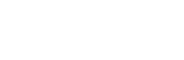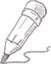### 实例

import numpy as np a = np.array([1,2,3,4]) b = np.array([10,20,30,40]) c = a * b print (c)

`[ 10  40  90 160]`

## 实例

import numpy as np a = np.array([[ 0, 0, 0], [10,10,10], [20,20,20], [30,30,30]])b = np.array([1,2,3])print(a + b)

```[[ 1  2  3]
[11 12 13]
[21 22 23]
[31 32 33]]```4x3 的二维数组与长为 3 的一维数组相加，等效于把数组 b 在二维上重复 4 次再运算：

## 实例

import numpy as np a = np.array([[ 0, 0, 0], [10,10,10], [20,20,20], [30,30,30]])b = np.array([1,2,3])bb = np.tile(b, (4, 1)) # 重复 b 的各个维度print(a + bb)

```[[ 1  2  3]
[11 12 13]
[21 22 23]
[31 32 33]]```

• 让所有输入数组都向其中形状最长的数组看齐，形状中不足的部分都通过在前面加 1 补齐。

• 输出数组的形状是输入数组形状的各个维度上的最大值。

• 如果输入数组的某个维度和输出数组的对应维度的长度相同或者其长度为 1 时，这个数组能够用来计算，否则出错。

• 当输入数组的某个维度的长度为 1 时，沿着此维度运算时都用此维度上的第一组值。

• 数组拥有相同形状。

• 当前维度的值相等。

• 当前维度的值有一个是 1。

29.9467 1 0 关注作者 收藏

## 评论(0)